Test: Fourier Series, Numerical Methods & Complex Variables- 2

# Test: Fourier Series, Numerical Methods & Complex Variables- 2

Test Description

## 30 Questions MCQ Test GATE Mechanical (ME) 2023 Mock Test Series | Test: Fourier Series, Numerical Methods & Complex Variables- 2

Test: Fourier Series, Numerical Methods & Complex Variables- 2 for Civil Engineering (CE) 2023 is part of GATE Mechanical (ME) 2023 Mock Test Series preparation. The Test: Fourier Series, Numerical Methods & Complex Variables- 2 questions and answers have been prepared according to the Civil Engineering (CE) exam syllabus.The Test: Fourier Series, Numerical Methods & Complex Variables- 2 MCQs are made for Civil Engineering (CE) 2023 Exam. Find important definitions, questions, notes, meanings, examples, exercises, MCQs and online tests for Test: Fourier Series, Numerical Methods & Complex Variables- 2 below.
Solutions of Test: Fourier Series, Numerical Methods & Complex Variables- 2 questions in English are available as part of our GATE Mechanical (ME) 2023 Mock Test Series for Civil Engineering (CE) & Test: Fourier Series, Numerical Methods & Complex Variables- 2 solutions in Hindi for GATE Mechanical (ME) 2023 Mock Test Series course. Download more important topics, notes, lectures and mock test series for Civil Engineering (CE) Exam by signing up for free. Attempt Test: Fourier Series, Numerical Methods & Complex Variables- 2 | 30 questions in 90 minutes | Mock test for Civil Engineering (CE) preparation | Free important questions MCQ to study GATE Mechanical (ME) 2023 Mock Test Series for Civil Engineering (CE) Exam | Download free PDF with solutions
 1 Crore+ students have signed up on EduRev. Have you?
Test: Fourier Series, Numerical Methods & Complex Variables- 2 - Question 1

### Choose the function f(t); –∞ < t < ∞, for which a Fourier series cannot be defined.

Detailed Solution for Test: Fourier Series, Numerical Methods & Complex Variables- 2 - Question 1

Fourier series is defined for periodic function and constant.

Fourier series can’t be defined for non-periodic functions.

The sum of two periodic function is also a periodic function.

3 sin (25 t) is a periodic function

4 cos (20 t + 3) and 2 sin (710 t) both are periodic function. So, 4 cos (20t + 3) + 2 sin (710 t) is also a periodic function.

e-|t| sin (25 t) is not a periodic function. So, Fourier series is can’t be defined.

1 is a constant. So, Fourier series is defined.

Test: Fourier Series, Numerical Methods & Complex Variables- 2 - Question 2

### The trigonometric Fourier series for the waveform f(t) shown below contains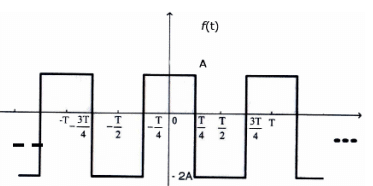Detailed Solution for Test: Fourier Series, Numerical Methods & Complex Variables- 2 - Question 2

From figure it’s an even function. so only cosine terms are present in the series and for DC  value,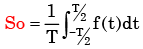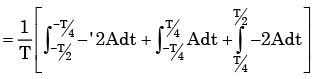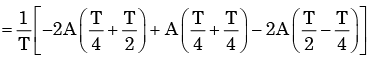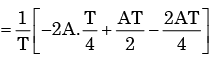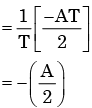So DC take negative value.

Test: Fourier Series, Numerical Methods & Complex Variables- 2 - Question 3

### Which of the following functions would have only odd powers of x in its Taylor series expansion about the point x = 0?

Detailed Solution for Test: Fourier Series, Numerical Methods & Complex Variables- 2 - Question 3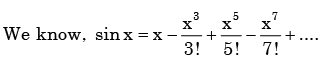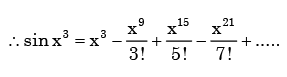Test: Fourier Series, Numerical Methods & Complex Variables- 2 - Question 4

The Taylor series expansion of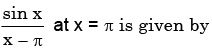Detailed Solution for Test: Fourier Series, Numerical Methods & Complex Variables- 2 - Question 4

We know.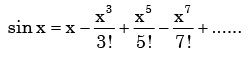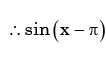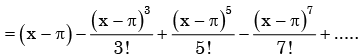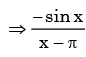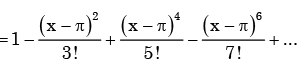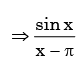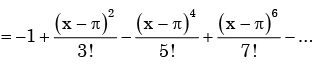Test: Fourier Series, Numerical Methods & Complex Variables- 2 - Question 5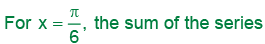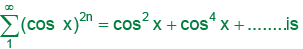Detailed Solution for Test: Fourier Series, Numerical Methods & Complex Variables- 2 - Question 5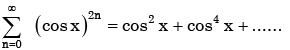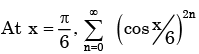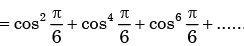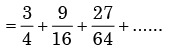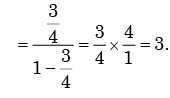Test: Fourier Series, Numerical Methods & Complex Variables- 2 - Question 6

The sum of the infinite series,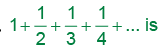Detailed Solution for Test: Fourier Series, Numerical Methods & Complex Variables- 2 - Question 6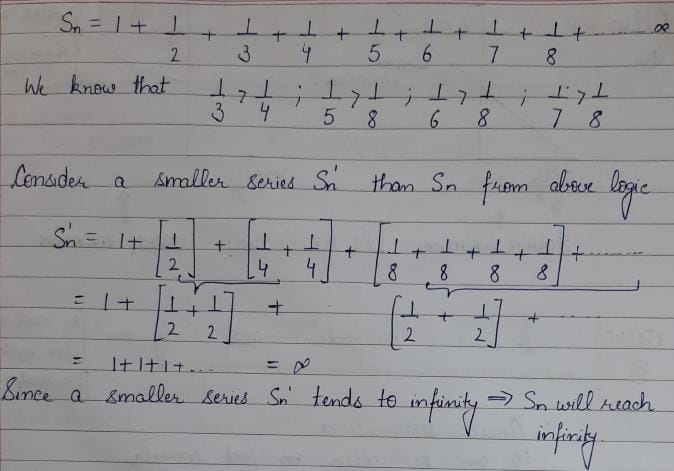Test: Fourier Series, Numerical Methods & Complex Variables- 2 - Question 7

The summation of seriesDetailed Solution for Test: Fourier Series, Numerical Methods & Complex Variables- 2 - Question 7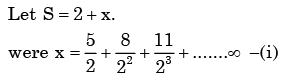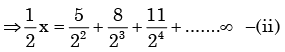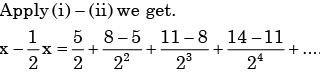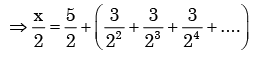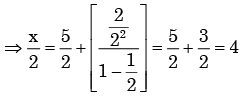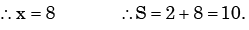Test: Fourier Series, Numerical Methods & Complex Variables- 2 - Question 8

Fourier series for the waveform, f (t) shown in fig. is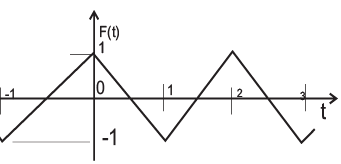Detailed Solution for Test: Fourier Series, Numerical Methods & Complex Variables- 2 - Question 8

From the figure, we say f (x) is even functions. so choice (c) is correct.

Test: Fourier Series, Numerical Methods & Complex Variables- 2 - Question 9

The Fourier Series coefficients, of a periodic signal x (t), expressed as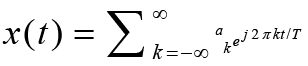are given by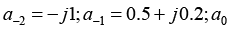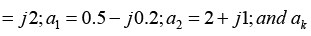Test: Fourier Series, Numerical Methods & Complex Variables- 2 - Question 10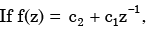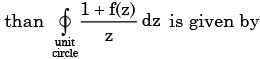Detailed Solution for Test: Fourier Series, Numerical Methods & Complex Variables- 2 - Question 10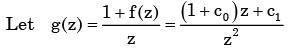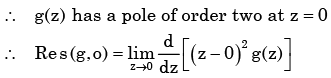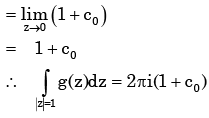Test: Fourier Series, Numerical Methods & Complex Variables- 2 - Question 11

For the function of a complex variable W = ln Z (where, W = u + jv and Z = x + jy), the u = constant lines get mapped in Z-plane as

Detailed Solution for Test: Fourier Series, Numerical Methods & Complex Variables- 2 - Question 11

Given,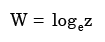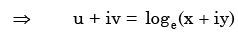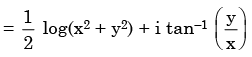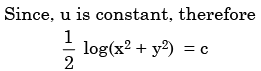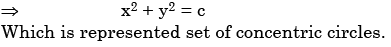Test: Fourier Series, Numerical Methods & Complex Variables- 2 - Question 12

ii, where i = √−1, is given by

Detailed Solution for Test: Fourier Series, Numerical Methods & Complex Variables- 2 - Question 12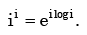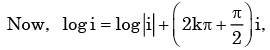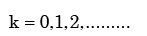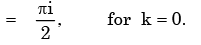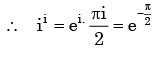Test: Fourier Series, Numerical Methods & Complex Variables- 2 - Question 13

Assuming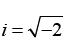and t is a real number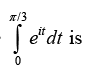Detailed Solution for Test: Fourier Series, Numerical Methods & Complex Variables- 2 - Question 13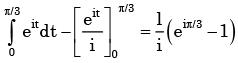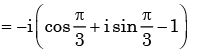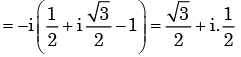Test: Fourier Series, Numerical Methods & Complex Variables- 2 - Question 14

The modulus of the complex number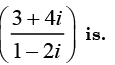Detailed Solution for Test: Fourier Series, Numerical Methods & Complex Variables- 2 - Question 14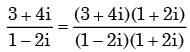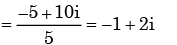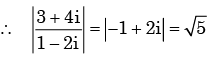Test: Fourier Series, Numerical Methods & Complex Variables- 2 - Question 15

Using Cauchy’s integral theorem, the value of the integral (integration being taken in counter clockwise direction)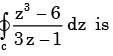Detailed Solution for Test: Fourier Series, Numerical Methods & Complex Variables- 2 - Question 15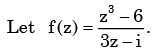Here f (z) has a singularities at z i / 3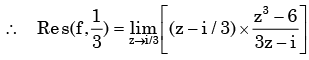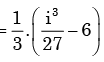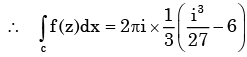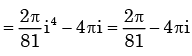Test: Fourier Series, Numerical Methods & Complex Variables- 2 - Question 16

Which one of the following is NOT true for complex number Z1and Z2

Detailed Solution for Test: Fourier Series, Numerical Methods & Complex Variables- 2 - Question 16

(a) is true since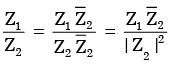(b) is true by triangle inequality of complex number.

(c) is not true since  |Z1 − Z2 |≥|Z1 |– |Z2

(d) is true since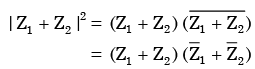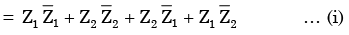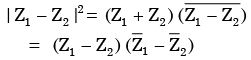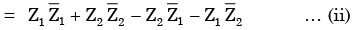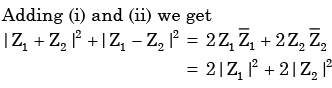Test: Fourier Series, Numerical Methods & Complex Variables- 2 - Question 17

For the equation, s3 - 4s2 + s + 6 =0

The number of roots in the left half of s-plane will be

Detailed Solution for Test: Fourier Series, Numerical Methods & Complex Variables- 2 - Question 17

Constructing Routh-array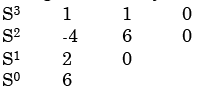Number of sign changes in the first column is two, therefore the number of roots in the left half splane is 2

Test: Fourier Series, Numerical Methods & Complex Variables- 2 - Question 18

The value of the integral of the complex function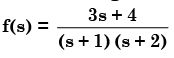Along the path |s| = 3 is

Detailed Solution for Test: Fourier Series, Numerical Methods & Complex Variables- 2 - Question 18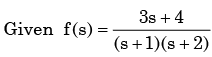f (s) has singularities at s =−1, −2 which are inside the given circle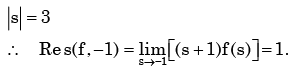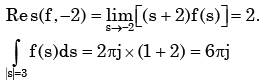Test: Fourier Series, Numerical Methods & Complex Variables- 2 - Question 19

For the function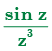of a complex variable z, the point z = 0 is

Detailed Solution for Test: Fourier Series, Numerical Methods & Complex Variables- 2 - Question 19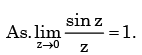Therefore the function has z = 0 is a pole of order 2.

Test: Fourier Series, Numerical Methods & Complex Variables- 2 - Question 20

The polynomial p(x) = x5 + x + 2 has

Detailed Solution for Test: Fourier Series, Numerical Methods & Complex Variables- 2 - Question 20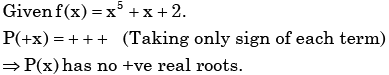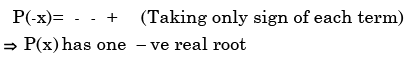As,  P(x) of degree 5  .So other four roots are complex.

Test: Fourier Series, Numerical Methods & Complex Variables- 2 - Question 21

If z = x + jy, where x and y are real, the value of |ejz| is

Detailed Solution for Test: Fourier Series, Numerical Methods & Complex Variables- 2 - Question 21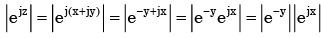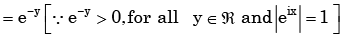Test: Fourier Series, Numerical Methods & Complex Variables- 2 - Question 22

The root mean squared value of x(t) = 3 + 2 sin (t) cos (2t) is

Detailed Solution for Test: Fourier Series, Numerical Methods & Complex Variables- 2 - Question 22

x(t) = 3 + 2 sin t cos 2t

x(t) = 3 + sin 3t – sin t

∴ Root mean square value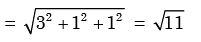Test: Fourier Series, Numerical Methods & Complex Variables- 2 - Question 23

We wish to solve x2 – 2 = 0 by Netwon Raphson technique. Let the initial guess b x0 = 1.0 Subsequent estimate of x(i.e.x1) will be:

Detailed Solution for Test: Fourier Series, Numerical Methods & Complex Variables- 2 - Question 23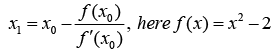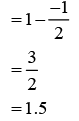Test: Fourier Series, Numerical Methods & Complex Variables- 2 - Question 24

The order of error is the Simpson’s rule for numerical integration with a step size h is

Test: Fourier Series, Numerical Methods & Complex Variables- 2 - Question 25

The table below gives values of a function F(x) obtained for values of x at intervals of 0.25.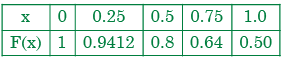The value of the integral of the function between the limits 0 to 1 using Simpson’s rule is

Detailed Solution for Test: Fourier Series, Numerical Methods & Complex Variables- 2 - Question 25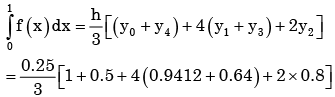Test: Fourier Series, Numerical Methods & Complex Variables- 2 - Question 26

A differential equation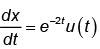has to be solved using trapezoidal rule of integration with a step size h=0.01s. Function u(t) indicates a unit step function. If x(0-)=0, then value of x at t=0.01s will be given by

Test: Fourier Series, Numerical Methods & Complex Variables- 2 - Question 27

Consider the series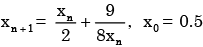obtained from the Newton-Raphson method. The series converges to

Detailed Solution for Test: Fourier Series, Numerical Methods & Complex Variables- 2 - Question 27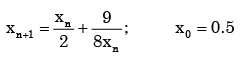The series converges when X n+1= Xn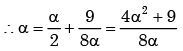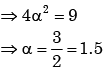Test: Fourier Series, Numerical Methods & Complex Variables- 2 - Question 28

Given a>0, we wish to calculate its reciprocal value 1/a by using Newton-Raphson method :
for f(x) = 0. For a=7 and starting wkith x0 = 0.2. the first 2 iterations will be

Detailed Solution for Test: Fourier Series, Numerical Methods & Complex Variables- 2 - Question 28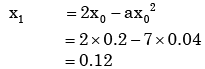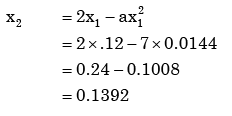Test: Fourier Series, Numerical Methods & Complex Variables- 2 - Question 29

With a 1 unit change in b, what is the change in x in the solution of the system of equations x + y = 2, 1.01 x + 0.99 y = b?

Detailed Solution for Test: Fourier Series, Numerical Methods & Complex Variables- 2 - Question 29

Given  x + y = 2               …………….. (i)

1.01 x + 0.99 y = b      …………….. (ii)

Multiply 0.99 is equation (i), and subtract from equation (ii), we get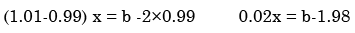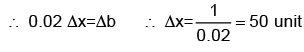Test: Fourier Series, Numerical Methods & Complex Variables- 2 - Question 30

The accuracy of Simpson's rule quadrature for a step size h is

## GATE Mechanical (ME) 2023 Mock Test Series

27 docs|243 tests
 Use Code STAYHOME200 and get INR 200 additional OFF Use Coupon Code
Information about Test: Fourier Series, Numerical Methods & Complex Variables- 2 Page
In this test you can find the Exam questions for Test: Fourier Series, Numerical Methods & Complex Variables- 2 solved & explained in the simplest way possible. Besides giving Questions and answers for Test: Fourier Series, Numerical Methods & Complex Variables- 2, EduRev gives you an ample number of Online tests for practice

## GATE Mechanical (ME) 2023 Mock Test Series

27 docs|243 tests